Test: Recursion- 2

# Test: Recursion- 2

Test Description

## 20 Questions MCQ Test GATE Computer Science Engineering(CSE) 2023 Mock Test Series | Test: Recursion- 2

Test: Recursion- 2 for Computer Science Engineering (CSE) 2023 is part of GATE Computer Science Engineering(CSE) 2023 Mock Test Series preparation. The Test: Recursion- 2 questions and answers have been prepared according to the Computer Science Engineering (CSE) exam syllabus.The Test: Recursion- 2 MCQs are made for Computer Science Engineering (CSE) 2023 Exam. Find important definitions, questions, notes, meanings, examples, exercises, MCQs and online tests for Test: Recursion- 2 below.
Solutions of Test: Recursion- 2 questions in English are available as part of our GATE Computer Science Engineering(CSE) 2023 Mock Test Series for Computer Science Engineering (CSE) & Test: Recursion- 2 solutions in Hindi for GATE Computer Science Engineering(CSE) 2023 Mock Test Series course. Download more important topics, notes, lectures and mock test series for Computer Science Engineering (CSE) Exam by signing up for free. Attempt Test: Recursion- 2 | 20 questions in 60 minutes | Mock test for Computer Science Engineering (CSE) preparation | Free important questions MCQ to study GATE Computer Science Engineering(CSE) 2023 Mock Test Series for Computer Science Engineering (CSE) Exam | Download free PDF with solutions
 1 Crore+ students have signed up on EduRev. Have you?
Test: Recursion- 2 - Question 1

### Predict output of following program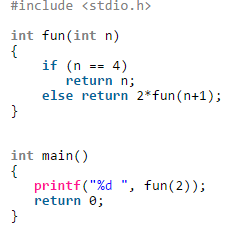Detailed Solution for Test: Recursion- 2 - Question 1

Fun(2)
|
2*fun(3)
|
2*fun(4)
|
4 After tree evaluation we get 16
So, C is the correct answer

Test: Recursion- 2 - Question 2

### Consider the following recursive function fun(x, y). What is the value of fun(4, 3)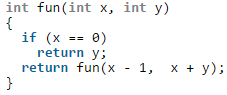Detailed Solution for Test: Recursion- 2 - Question 2

The function fun() calculates and returns ((1 + 2 … + x-1 + x) +y) which is x(x+1)/2 + y.

Test: Recursion- 2 - Question 3

### What does the following function print for n = 25?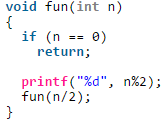Detailed Solution for Test: Recursion- 2 - Question 3

The function mainly prints binary representation in reverse order.

Test: Recursion- 2 - Question 4

What does the following function do?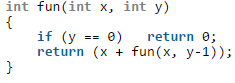Detailed Solution for Test: Recursion- 2 - Question 4

The function adds x to itself y times which is x*y.

Test: Recursion- 2 - Question 5

What does fun2() do in general?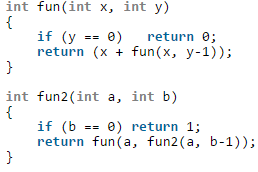Detailed Solution for Test: Recursion- 2 - Question 5

The function multiplies x to itself y times which is xy.

Test: Recursion- 2 - Question 6

Output of following program?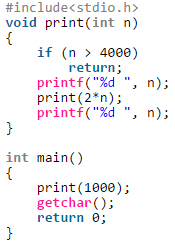Test: Recursion- 2 - Question 7

What does the following function do?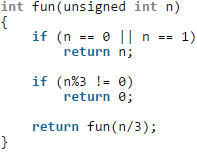Test: Recursion- 2 - Question 8

Predict the output of following program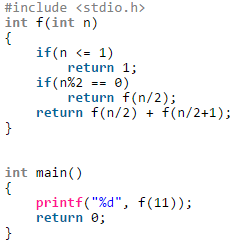Test: Recursion- 2 - Question 9

Consider the following recursive C function that takes two arguments

unsigned int foo(unsigned int n, unsigned int r){

if(n >0) return (n%r + foo (n/r, r));

else return 0;

}

Q.
What is the return value of the function foo when it is called as foo(345, 10) ?

Detailed Solution for Test: Recursion- 2 - Question 9

The call foo(345, 10) returns sum of decimal digits (because r is 10) in the number n. Sum of digits for 345 is 3 + 4 + 5 = 12.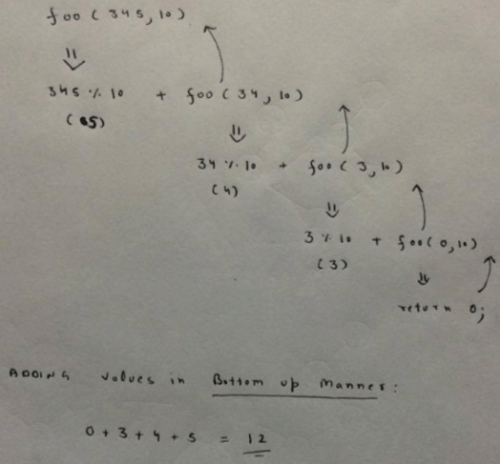Test: Recursion- 2 - Question 10

Consider the same recursive C function that takes two arguments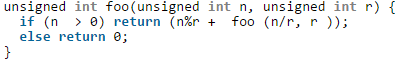Q. What is the return value of the function foo when it is called as foo(513, 2)?

Detailed Solution for Test: Recursion- 2 - Question 10

foo(513, 2) will return 1 + foo(256, 2). All subsequent recursive calls (including foo(256, 2)) will return 0 + foo(n/2, 2) except the last call foo(1, 2). The last call foo(1, 2) returns 1. So, the value returned by foo(513, 2) is 1 + 0 + 0…. + 0 + 1. The function foo(n, 2) basically returns sum of bits (or count of set bits) in the number n.

Test: Recursion- 2 - Question 11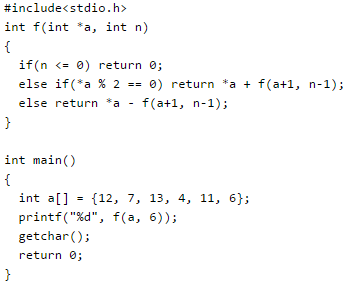Detailed Solution for Test: Recursion- 2 - Question 11

f() is a recursive function which adds f(a+1, n-1) to *a if *a is even. If *a is odd then f() subtracts f(a+1, n-1) from *a. See below recursion tree for execution of f(a, 6).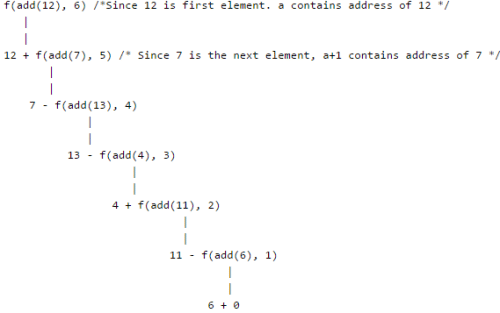So, the final returned value is 12 + (7 – (13 – (4 + (11 – (6 + 0))))) = 15

Test: Recursion- 2 - Question 12

Output of following program?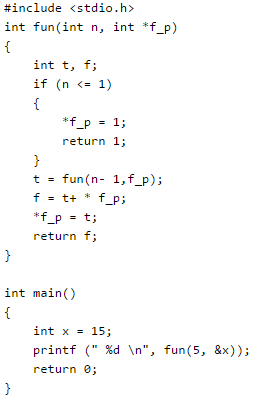Detailed Solution for Test: Recursion- 2 - Question 12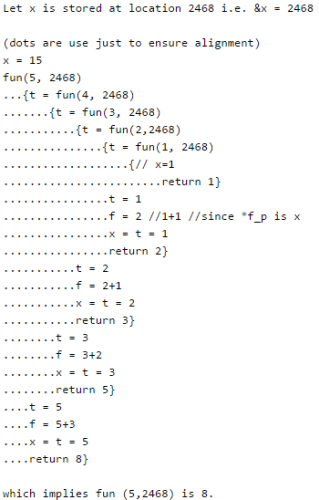Test: Recursion- 2 - Question 13

Consider the following code snippet: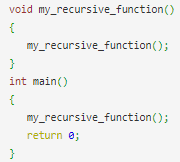What will happen when the above snippet is executed?

Detailed Solution for Test: Recursion- 2 - Question 13

Every function call is stored in the stack memory. In this case, there is no terminating condition(base case). So, my_recursive_function() will be called continuously till the stack overflows and there is no more space to store the function calls. At this point of time, the program will stop abruptly.

Test: Recursion- 2 - Question 14

Consider the C function given below.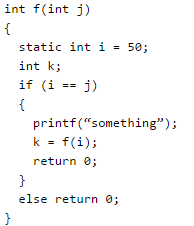Q. Which one of the following is TRUE?

Detailed Solution for Test: Recursion- 2 - Question 14

When j is 50, the function would call itself again and again as neither i nor j is changed inside the recursion.

Test: Recursion- 2 - Question 15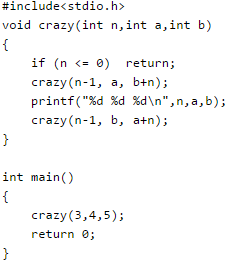Test: Recursion- 2 - Question 16

Consider the following C function: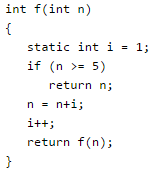The value returned by f(1) is

Test: Recursion- 2 - Question 17

Consider the following C function.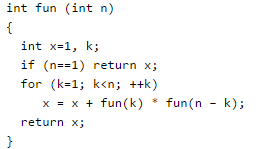Q. The return value of fun(5) is __________.

Detailed Solution for Test: Recursion- 2 - Question 17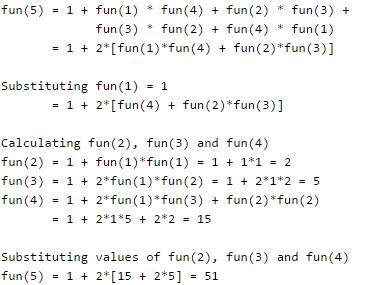Test: Recursion- 2 - Question 18

Consider the following recursive C function. If get(6) function is being called in main() then how many times will the get() function be invoked before returning to the main()?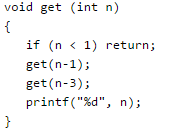Detailed Solution for Test: Recursion- 2 - Question 18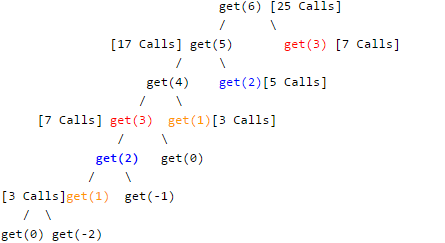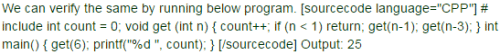Test: Recursion- 2 - Question 19

What will be the output of the following C program?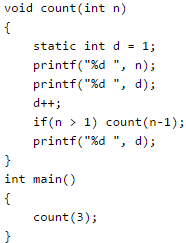Detailed Solution for Test: Recursion- 2 - Question 19

count(3) will print value of n and d. So 3 1 will be printed and d will become 2.
Then count(2) will be called. It will print value of n and d.
So 2 2 will be printed and d will become 3.
Then count(1) will be called. It will print value of n and d.
So 1 3 will be printed and d will become 4.
Now count(1) will print value of d which is 4. count(1) will finish its execution.
Then count(2) will print value of d which is 4.
Similarly, count(3) will print value of d which is 4.
So series will be A.

Test: Recursion- 2 - Question 20

The function f is defined as follows: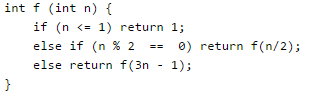Assuming that arbitrarily large integers can be passed as a parameter to the function, consider the following statements.
1. The function f terminates for finitely many different values of n ≥ 1.
ii. The function f terminates for infinitely many different values of n ≥ 1.
iii. The function f does not terminate for finitely many different values of n ≥ 1.
iv. The function f does not terminate for infinitely many different values of n ≥ 1.
Which one of the following options is true of the above?

Detailed Solution for Test: Recursion- 2 - Question 20

The function terminates for all values having a factor of 2 {(2.x)2==0}
So, (i) is false and (ii) is TRUE.
Let n = 3, it will terminate in 2nd iteration.
Let n=5, it will go like 5 - 14 - 7 - 20 - 10 - 5 – and now it will repeat.
And any number with a factor of 5 and 2, there are infinite recursions possible.
So, (iv) is TRUE and (iii) is false.

## GATE Computer Science Engineering(CSE) 2023 Mock Test Series

150 docs|215 tests
Information about Test: Recursion- 2 Page
In this test you can find the Exam questions for Test: Recursion- 2 solved & explained in the simplest way possible. Besides giving Questions and answers for Test: Recursion- 2, EduRev gives you an ample number of Online tests for practice

## GATE Computer Science Engineering(CSE) 2023 Mock Test Series

150 docs|215 tests(Scan QR code)